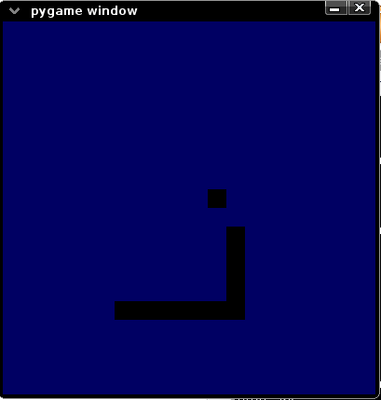# AttoSnake - 496 bytes -- Final?

A Snake game which aims to be as small in filesize as possible, while retaining fun and playability.Jordan Trudgett
(tgfcoder)
```It's still just as playable! The input is tidy, not
delayed, and fun to play with and best of all, there are NO
glitches (that I'm aware of.) To quit, just chuck in a
KeyboardInterrupt or crash into yourself. It WILL NOT quit
neatly in IDLE, unfortunately. It was a tradeoff for less
bytes. Enjoy! For other snakers: use & lt; and & rt; for
angular brackets (no spaces after &) Use this to make your
code HTML friendly!

Apologies: pygame thought some of the angular brackets were
tags.

FIXED NOW. The code you see below can be copied and pasted into your favourite editor and run as a python file as-is.

Thanks for playing!
```

### Changes

```import pygame as p,random
I=random.randint
p.init()
d=p.display
s=d.set_mode(*2)
B=[]
P=0
L=5
e=I(0,70)
Y=p.Rect(0,0,8,8)
Q=s.fill
l=lambda z:(8*(z%9),8*(z/9))
m=lambda n:Q(0,Y.move(l(n)))
S=[-1,1,0,0]
N=1
t=9
while 1:
Q(99)
m(e)
P=P+t
B+=[P]
B=B[-L:]
map(m,B)
if P==e:L+=1;e=I(0,70)
if P in B[:-1] or P%9==8 or P&256or P>71:Z
d.flip()
p.time.wait(99)
o=N
for v in p.event.get():
if v.type==2:
N=v.key-273
if -1<N-2+o<3:t=S[::-1][N]+S[N]*9
```